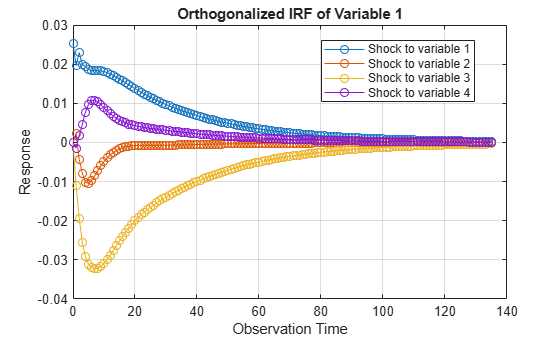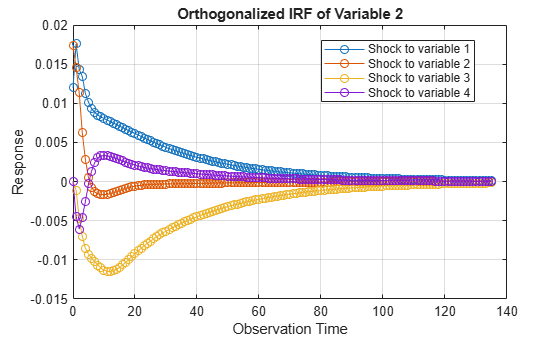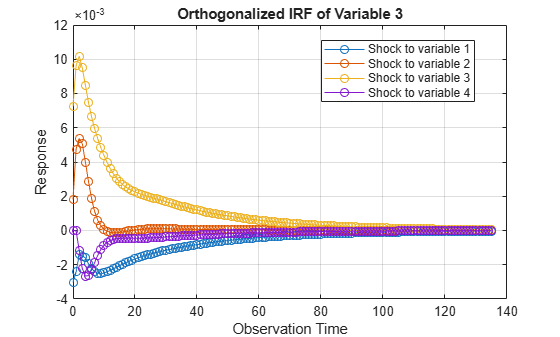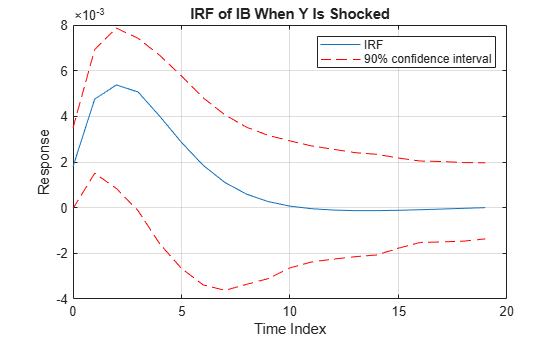# irf

Generate vector autoregression (VAR) model impulse responses

## Syntax

``Response = irf(Mdl)``
``Response = irf(Mdl,Name,Value)``
``[Response,Lower,Upper] = irf(___)``

## Description

The `irf` function returns the dynamic response, or the impulse response function (IRF), to a one-standard-deviation shock to each variable in a VAR(p) model. A fully specified `varm` model object characterizes the VAR model.

To estimate or plot the IRF of a dynamic linear model characterized by structural, autoregression, or moving average coefficient matrices, see `armairf`.

IRFs trace the effects of an innovation shock to one variable on the response of all variables in the system. In contrast, the forecast error variance decomposition (FEVD) provides information about the relative importance of each innovation in affecting all variables in the system. To estimate the FEVD of a VAR model characterized by a `varm` model object, see `fevd`.

example

````Response = irf(Mdl)` returns the 20-period, orthogonalized IRF of the response variables that compose the VAR(p) model `Mdl`, characterized by a fully specified `varm` model object. `irf` shocks variables at time 0, and returns the IRF for times 0 through 19.```

example

````Response = irf(Mdl,Name,Value)` uses additional options specified by one or more name-value pair arguments. For example, `'NumObs',10,'Method',"generalized"` specifies estimating a generalized IRF for 10 time points starting at time 0, during which `irf` applies the shock, and ending at period 9.```

example

````[Response,Lower,Upper] = irf(___)` uses any of the input argument combinations in the previous syntaxes and returns lower and upper 95% confidence bounds for each period and variable in the IRF: If you specify series of residuals by using the `E` name-value pair argument, then `irf` estimates the confidence bounds by bootstrapping the specified residuals.Otherwise, `irf` estimates confidence bounds by conducting Monte Carlo simulation. If `Mdl` is a custom `varm` model object (an object not returned by `estimate` or modified after estimation), `irf` might require a sample size for the simulation `SampleSize` or presample responses `Y0`.```

## Examples

collapse all

Fit a 4-D VAR(2) model to Danish money and income rate series. Then, estimate and plot the orthogonalized IRF from the estimated model.

Load the Danish money and income data set.

`load Data_JDanish`

The data set includes four time series in the table `DataTable`. For more details on the data set, enter `Description` at the command line.

Assuming that the series are stationary, create a `varm` model object that represents a 4-D VAR(2) model. Specify the variable names.

```Mdl = varm(4,2); Mdl.SeriesNames = DataTable.Properties.VariableNames;```

`Mdl` is a `varm` model object specifying the structure of a 4-D VAR(2) model; it is a template for estimation.

Fit the VAR(2) model to the data set.

`Mdl = estimate(Mdl,DataTable.Series);`

`Mdl` is a fully specified `varm` model object representing an estimated 4-D VAR(2) model.

Estimate the orthogonalized IRF from the estimated VAR(2) model.

`Response = irf(Mdl);`

`Response` is a 20-by-4-by-4 array representing the IRF of `Mdl`. Rows correspond to consecutive time points from time 0 to 19, columns correspond to variables receiving a one-standard-deviation innovation shock at time 0, and pages correspond to responses of variables to the variable being shocked. `Mdl.SeriesNames` specifies the variable order.

Display the IRF of the bond rate (variable 3, `IB`) when the log of real income (variable 2, `Y`) is shocked at time 0.

`Response(:,2,3)`
```ans = 20×1 0.0018 0.0048 0.0054 0.0051 0.0040 0.0029 0.0019 0.0011 0.0006 0.0003 ⋮ ```

Plot the IRFs of all series on separate plots by passing the estimated AR coefficient matrices and innovations covariance matrix of `Mdl` to `armairf`.

`armairf(Mdl.AR,[],"InnovCov",Mdl.Covariance);`Each plot shows the four IRFs of a variable when all other variables are shocked at time 0. `Mdl.SeriesNames` specifies the variable order.

Consider the 4-D VAR(2) model in Estimate and Plot VAR Model IRF. Estimate the generalized IRF of the system for 50 periods.

Load the Danish money and income data set, then estimate the VAR(2) model.

```load Data_JDanish Mdl = varm(4,2); Mdl.SeriesNames = DataTable.Properties.VariableNames; Mdl = estimate(Mdl,DataTable.Series);```

Estimate the generalized IRF from the estimated VAR(2) model.

`Response = irf(Mdl,"Method","generalized","NumObs",50);`

`Response` is a 50-by-4-by-4 array representing the generalized IRF of `Mdl`.

Plot the generalized IRF of the bond rate when real income is shocked at time 0.

```figure; plot(0:49,Response(:,2,3)) title("IRF of IB When Y Is Shocked") xlabel("Observation Time") ylabel("Response") grid on```The bond rate fades slowly when real income is shocked at time 0.

Consider the 4-D VAR(2) model in Estimate and Plot VAR Model IRF. Estimate and plot its orthogonalized IRF and 95% Monte Carlo confidence intervals on the true IRF.

Load the Danish money and income data set, then estimate the VAR(2) model.

```load Data_JDanish Mdl = varm(4,2); Mdl.SeriesNames = DataTable.Properties.VariableNames; Mdl = estimate(Mdl,DataTable.Series);```

Estimate the IRF and corresponding 95% Monte Carlo confidence intervals from the estimated VAR(2) model.

```rng(1); % For reproducibility [Response,Lower,Upper] = irf(Mdl);```

`Response`, `Lower`, and `Upper` are 20-by-4-by-4 arrays representing the orthogonalized IRF of `Mdl` and corresponding lower and upper bounds of the confidence intervals. For all arrays, rows correspond to consecutive time points from time 0 to 19, columns correspond to variables receiving a one-standard-deviation innovation shock at time 0, and pages correspond to responses of variables to the variable being shocked. `Mdl.SeriesNames` specifies the variable order.

Plot the orthogonalized IRF with its confidence bounds of the bond rate when real income is shocked at time 0.

```irfshock2resp3 = Response(:,2,3); IRFCIShock2Resp3 = [Lower(:,2,3) Upper(:,2,3)]; figure; h1 = plot(0:19,irfshock2resp3); hold on h2 = plot(0:19,IRFCIShock2Resp3,'r--'); legend([h1 h2(1)],["IRF" "95% Confidence Interval"]) xlabel("Time Index"); ylabel("Response"); title("IRF of IB When Y Is Shocked"); grid on hold off```The effect of the impulse to real income on the bond rate fades after 10 periods.

Consider the 4-D VAR(2) model in Estimate and Plot VAR Model IRF. Estimate and plot its orthogonalized IRF and 90% bootstrap confidence intervals on the true IRF.

Load the Danish money and income data set, then estimate the VAR(2) model. Return the residuals from model estimation.

```load Data_JDanish Mdl = varm(4,2); Mdl.SeriesNames = DataTable.Properties.VariableNames; [Mdl,~,~,E] = estimate(Mdl,DataTable.Series); T = size(DataTable,1) % Total sample size```
```T = 55 ```
`n = size(E,1) % Effective sample size`
```n = 53 ```

`E` is a 53-by-4 array of residuals. Columns correspond to the variables in `Mdl.SeriesNames`. The `estimate` function requires `Mdl.P` = 2 observations to initialize a VAR(2) model for estimation. Because presample data (`Y0`) is unspecified, `estimate` takes the first two observations in the specified response data to initialize the model. Therefore, the resulting effective sample size is `T``Mdl.P` = 53, and rows of `E` correspond to the observation indices 3 through `T`.

Estimate the orthogonalized IRF and corresponding 90% bootstrap confidence intervals from the estimated VAR(2) model. Draw 500 paths of length `n` from the series of residuals.

```rng(1); % For reproducibility [Response,Lower,Upper] = irf(Mdl,"E",E,"NumPaths",500,... "Confidence",0.9);```

Plot the orthogonalized IRF with its confidence bounds of the bond rate when real income is shocked at time 0.

```irfshock2resp3 = Response(:,2,3); IRFCIShock2Resp3 = [Lower(:,2,3) Upper(:,2,3)]; figure; h1 = plot(0:19,irfshock2resp3); hold on h2 = plot(0:19,IRFCIShock2Resp3,'r--'); legend([h1 h2(1)],["IRF" "90% Confidence Interval"]) xlabel("Time Index"); ylabel("Response"); title("IRF of IB When Y Is Shocked"); grid on hold off```The effect of the impulse to real income on the bond rate fades after 10 periods.

## Input Arguments

collapse all

VAR model, specified as a `varm` model object created by `varm` or `estimate`. `Mdl` must be fully specified.

### Name-Value Arguments

Specify optional pairs of arguments as `Name1=Value1,...,NameN=ValueN`, where `Name` is the argument name and `Value` is the corresponding value. Name-value arguments must appear after other arguments, but the order of the pairs does not matter.

Before R2021a, use commas to separate each name and value, and enclose `Name` in quotes.

Example: `'NumObs',10,'Method',"generalized"` specifies estimating a generalized IRF for 10 time points starting at time 0, during which `irf` applies the shock, and ending at period 9.

Options for All IRFs

collapse all

Number of periods for which `irf` computes the IRF, specified as the comma-separated pair consisting of `'NumObs'` and a positive integer. `NumObs` specifies the number of observations to include in the IRF (the number of rows in `Response`).

Example: `'NumObs',10` specifies the inclusion of 10 time points in the IRF starting at time 0, during which `irf` applies the shock, and ending at period 9.

Data Types: `double`

IRF computation method, specified as the comma-separated pair consisting of `'Method'` and a value in this table.

ValueDescription
`"orthogonalized"`Compute impulse responses using orthogonalized, one-standard-deviation innovation shocks. `irf` uses the Cholesky factorization of `Mdl.Covariance` for orthogonalization.
`"generalized"`Compute impulse responses using one-standard-deviation innovation shocks.

Example: `'Method',"generalized"`

Data Types: `string`

Options for Confidence Bound Estimation

collapse all

Number of sample paths (trials) to generate, specified as the comma-separated pair consisting of `'NumPaths'` and a positive integer.

Example: `'NumPaths',1000` generates `1000` sample paths from which the software derives the confidence bounds.

Data Types: `double`

Number of observations for the Monte Carlo simulation or bootstrap per sample path, specified as the comma-separated pair consisting of `'SampleSize'` and a positive integer.

• If `Mdl` is an estimated `varm` model object (an object returned by `estimate` and unmodified thereafter), then the default is the sample size of the data to which the model is fit (see `summarize`).

• If `irf` estimates confidence bounds by conducting a Monte Carlo simulation (for details, see `E`), you must specify `SampleSize`.

• If `irf` estimates confidence bounds by bootstrapping residuals, the default is the length of the specified series of residuals (`size(E,1)`).

Example: If you specify `'SampleSize',100` and do not specify the `'E'` name-value pair argument, the software estimates confidence bounds from `NumPaths` random paths of length `100` from `Mdl`.

Example: If you specify `'SampleSize',100,'E',E`, the software resamples, with replacement, `100` observations (rows) from `E` to form a sample path of innovations to filter through `Mdl`. The software forms `NumPaths` random sample paths from which it derives confidence bounds.

Data Types: `double`

Presample response data that provides initial values for model estimation during the simulation, specified as the comma-separated pair consisting of `'Y0'` and a `numpreobs`-by-`numseries` numeric matrix.

Rows of `Y0` correspond to periods in the presample, and the last row contains the latest presample response. `numpreobs` is the number of specified presample responses and it must be at least `Mdl.P`. If `numpreobs` exceeds `Mdl.P`, then `irf` uses only the latest `Mdl.P` rows.

`numseries` is the dimensionality of the input VAR model `Mdl.NumSeries`. Columns must correspond to the response variables in `Mdl.SeriesNames`.

• If `Mdl` is an estimated `varm` model object (an object returned by `estimate` and unmodified thereafter), `irf` sets `Y0` to the presample response data used for estimation by default (see `'Y0'`).

• Otherwise, you must specify `Y0`.

Data Types: `double`

Predictor data for estimating the model regression component during the simulation, specified as the comma-separated pair consisting of `'X'` and a numeric matrix containing `numpreds` columns.

`numpreds` is the number of predictor variables (`size(Mdl.Beta,2)`).

Rows correspond to observations. `X` must have at least `SampleSize` rows. If you supply more rows than necessary, `irf` uses only the latest `SampleSize` observations. The last row contains the latest observation.

Columns correspond to individual predictor variables. All predictor variables are present in the regression component of each response equation.

To maintain model consistency when `irf` estimates the confidence bounds, a good practice is to specify `X` when `Mdl` has a regression component. If `Mdl` is an estimated model, specify the predictor data used during model estimation (see `'X'`).

By default, `irf` excludes the regression component from confidence bound estimation, regardless of its presence in `Mdl`.

Data Types: `double`

Series of residuals from which to draw bootstrap samples, specified as the comma-separated pair consisting of `'E'` and a numeric matrix containing `numseries` columns. `irf` assumes that `E` is free of serial correlation.

Columns contain the residual series corresponding to the response series names in `Mdl.SeriesNames`.

If `Mdl` is an estimated `varm` model object (an object returned by `estimate`), you can specify `E` as the inferred residuals from estimation (see `E` or `infer`).

By default, `irf` derives confidence bounds by conducting a Monte Carlo simulation.

Data Types: `double`

Confidence level for the confidence bounds, specified as a numeric scalar in the interval [0,1].

For each period, randomly drawn confidence intervals cover the true response `100*Confidence`% of the time.

The default value is `0.95`, which implies that the confidence bounds represent 95% confidence intervals.

Data Types: `double`

## Output Arguments

collapse all

IRF, returned as a `numobs`-by-`numseries`-by-`numseries` numeric array. `numobs` is the value of `NumObs`. Columns and pages correspond to the response variables in `Mdl.SeriesNames`.

`Response(t + 1,j,k)` is the impulse response of variable `k` to a one-standard-deviation innovation shock to variable `j` at time 0, for `t` = 0, 1, ..., `numObs` – 1, `j` = 1,2,...,`numseries`, and `k` = 1,2,...,`numseries`.

Lower confidence bounds, returned as a `numobs`-by-`numseries`-by-`numseries` numeric array. Elements of `Lower` correspond to elements of `Response`.

`Lower(t + 1,j,k)` is the lower bound of the `100*Confidence`% percentile interval on the true impulse response of variable `k` to a one-standard-deviation innovation shock to variable `j` at time 0.

Upper confidence bounds, returned as a `numobs`-by-`numseries`-by-`numseries` numeric array. Elements of `Upper` correspond to elements of `Response`.

`Upper(t + 1,j,k)` is the upper bound of the `100*Confidence`% percentile interval on the true impulse response of variable `k` to a one-standard-deviation innovation shock to variable `j` at time 0.

collapse all

### Impulse Response Function

An impulse response function (IRF) of a time series model (or dynamic response of the system) measures the changes in the future responses of all variables in the system when a variable is shocked by an impulse. In other words, the IRF at time t is the derivative of the responses at time t with respect to an innovation at time t0 (the time that innovation was shocked), tt0.

Consider a `numseries`-D VAR(p) model for the multivariate response variable yt. In lag operator notation, the infinite lag MA representation of yt is:

`$\begin{array}{c}{y}_{t}={\Phi }^{-1}\left(L\right)\left(c+\beta {x}_{t}+\delta t\right)+{\Phi }^{-1}\left(L\right){\epsilon }_{t}\\ =\Omega \left(L\right)\left(c+\beta {x}_{t}+\delta t\right)+\Omega \left(L\right){\epsilon }_{t}.\end{array}$`

The general form of the IRF of yt shocked by an impulse to variable j by one standard deviation of its innovation m periods into the future is:

`${\psi }_{j}\left(m\right)={C}_{m}{e}_{j}.$`

• ej is a selection vector of length `numseries` containing a 1 in element j and zeros elsewhere.

• For the orthogonalized IRF, ${C}_{m}={\Omega }_{m}P,$ where P is the lower triangular factor in the Cholesky factorization of Σ, and Ωm is the lag m coefficient of Ω(L).

• For the generalized IRF, ${C}_{m}={\sigma }_{j}^{-1}{\Omega }_{m}\Sigma ,$ where σj is the standard deviation of innovation j.

• The IRF is free of the model constant, regression component, and time trend.

### Vector Autoregression Model

A vector autoregression (VAR) model is a stationary multivariate time series model consisting of a system of m equations of m distinct response variables as linear functions of lagged responses and other terms.

A VAR(p) model in difference-equation notation and in reduced form is

`${y}_{t}=c+{\Phi }_{1}{y}_{t-1}+{\Phi }_{2}{y}_{t-2}+...+{\Phi }_{p}{y}_{t-p}+\beta {x}_{t}+\delta t+{\epsilon }_{t}.$`

• yt is a `numseries`-by-1 vector of values corresponding to `numseries` response variables at time t, where t = 1,...,T. The structural coefficient is the identity matrix.

• c is a `numseries`-by-1 vector of constants.

• Φj is a `numseries`-by-`numseries` matrix of autoregressive coefficients, where j = 1,...,p and Φp is not a matrix containing only zeros.

• xt is a `numpreds`-by-1 vector of values corresponding to `numpreds` exogenous predictor variables.

• β is a `numseries`-by-`numpreds` matrix of regression coefficients.

• δ is a `numseries`-by-1 vector of linear time-trend values.

• εt is a `numseries`-by-1 vector of random Gaussian innovations, each with a mean of 0 and collectively a `numseries`-by-`numseries` covariance matrix Σ. For ts, εt and εs are independent.

Condensed and in lag operator notation, the system is

`$\Phi \left(L\right){y}_{t}=c+\beta {x}_{t}+\delta t+{\epsilon }_{t},$`

where $\Phi \left(L\right)=I-{\Phi }_{1}L-{\Phi }_{2}{L}^{2}-...-{\Phi }_{p}{L}^{p}$, Φ(L)yt is the multivariate autoregressive polynomial, and I is the `numseries`-by-`numseries` identity matrix.

## Algorithms

• `NaN` values in `Y0`, `X`, and `E` indicate missing data. `irf` removes missing data from these arguments by list-wise deletion. Each argument, if a row contains at least one `NaN`, then `irf` removes the entire row.

List-wise deletion reduces the sample size, can create irregular time series, and can cause `E` and `X` to be unsynchronized.

• If `Method` is `"orthogonalized"`, then the resulting IRF depends on the order of the variables in the time series model. If `Method` is `"generalized"`, then the resulting IRF is invariant to the order of the variables. Therefore, the two methods generally produce different results.

• If `Mdl.Covariance` is a diagonal matrix, then the resulting generalized and orthogonalized IRFs are identical. Otherwise, the resulting generalized and orthogonalized IRFs are identical only when the first variable shocks all variables (that is, all else being the same, both methods yield the same value of `Response(:,1,:)`).

• The predictor data `X` represents a single path of exogenous multivariate time series. If you specify `X` and the VAR model `Mdl` has a regression component (`Mdl.Beta` is not an empty array), `irf` applies the same exogenous data to all paths used for confidence interval estimation.

• `irf` conducts a simulation to estimate the confidence bounds `Lower` and `Upper`.

• If you do not specify residuals `E`, then `irf` conducts a Monte Carlo simulation by following this procedure:

1. Simulate `NumPaths` response paths of length `SampleSize` from `Mdl`.

2. Fit `NumPaths` models that have the same structure as `Mdl` to the simulated response paths. If `Mdl` contains a regression component and you specify `X`, then `irf` fits the `NumPaths` models to the simulated response paths and `X` (the same predictor data for all paths).

3. Estimate `NumPaths` IRFs from the `NumPaths` estimated models.

4. For each time point t = 0,…,`NumObs`, estimate the confidence intervals by computing 1 – `Confidence` and `Confidence` quantiles (upper and lower bounds, respectively).

• If you specify residuals `E`, then `irf` conducts a nonparametric bootstrap by following this procedure:

1. Resample, with replacement, `SampleSize` residuals from `E`. Perform this step `NumPaths` times to obtain `NumPaths` paths.

2. Center each path of bootstrapped residuals.

3. Filter each path of centered, bootstrapped residuals through `Mdl` to obtain `NumPaths` bootstrapped response paths of length `SampleSize`.

4. Complete steps 2 through 4 of the Monte Carlo simulation, but replace the simulated response paths with the bootstrapped response paths.

 Hamilton, James D. Time Series Analysis. Princeton, NJ: Princeton University Press, 1994.

 Lütkepohl, Helmut. New Introduction to Multiple Time Series Analysis. New York, NY: Springer-Verlag, 2007.

 Pesaran, H. H., and Y. Shin. "Generalized Impulse Response Analysis in Linear Multivariate Models." Economic Letters. Vol. 58, 1998, pp. 17–29.